## A CD is spinning on a CD player. In 12 radians, the cd has reached an angular speed of 17 r a d s by accelerating with a constant accelerati

Question

A CD is spinning on a CD player. In 12 radians, the cd has reached an angular speed of 17 r a d s by accelerating with a constant acceleration of 3 r a d s 2 . What was the initial angular speed of the CD

in progress 0
7 months 2021-07-12T11:45:28+00:00 1 Answers 2 views 0

## Answers ( )

The initial angular speed of the CD is equal to 14.73 rad/s.

Explanation:

Given that,

Angular displacement,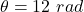Final angular speed,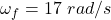The acceleration of the CD,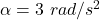We need to find the initial angular speed of the CD. Using third equation of kinematics to find it such that,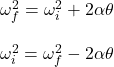Put all the values,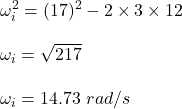So, the initial angular speed of the CD is equal to 14.73 rad/s.